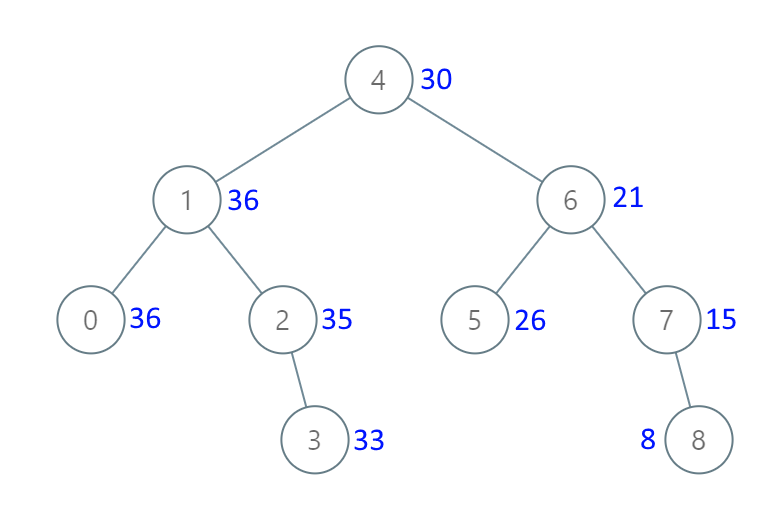1038. Binary Search Tree to Greater Sum Tree

#### QUESTION:

Given the root of a binary search tree with distinct values, modify it so that every node has a new value equal to the sum of the values of the original tree that are greater than or equal to node.val.

As a reminder, a binary search tree is a tree that satisfies these constraints:

The left subtree of a node contains only nodes with keys less than the node’s key. The right subtree of a node contains only nodes with keys greater than the node’s key. Both the left and right subtrees must also be binary search trees.

Example 1:Input: [4,1,6,0,2,5,7,null,null,null,3,null,null,null,8] Output: [30,36,21,36,35,26,15,null,null,null,33,null,null,null,8]

Note:

The number of nodes in the tree is between 1 and 100. Each node will have value between 0 and 100. The given tree is a binary search tree.

#### SOLUTION:

``````class Solution {
public TreeNode bstToGst(TreeNode root) {
bstToGstHelper(root);
return root;
}
public int bstToGstSum = 0;
public void bstToGstHelper(TreeNode root) {
if(root == null) return;
if(root.right!=null) bstToGstHelper(root.right);
bstToGstSum+=root.val;
root.val = bstToGstSum;
if(root.left!=null) bstToGstHelper(root.left);
}
}
``````
>